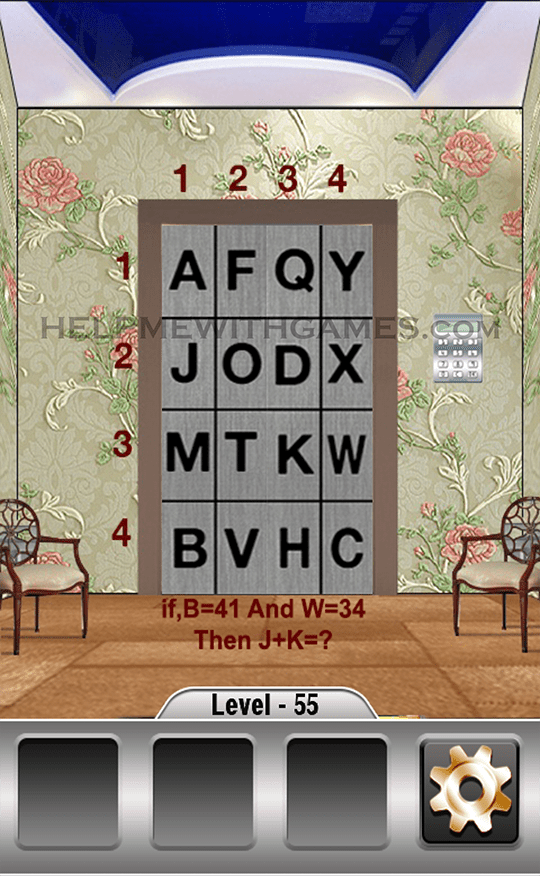# 100 Weird Doors – Walkthrough – Level 55100 Weird Doors Impossible & Unravel Escape Puzzle is developed by Fun Games Tree

All the answers here! The main goal in 100 Weird Doors is to escape the room.
Using all the possibilities of the device, you need to open each of the 100 doors using your brain and imagination.
Search for hidden objects and solve puzzles to make it through each doors!

Solution for the level 55:

B=41 because the number on the left is 4 and the top one is 1
W=34 because the number on the left is 3 and the top one is 4
So,
J=21 because the number on the left is 2 and the top one is 1
K=33 because the number on the left is 3 and the top one is 3
21+33=54
The code is 54Click here to go back to the list of all the levels

This site uses Akismet to reduce spam. Learn how your comment data is processed.